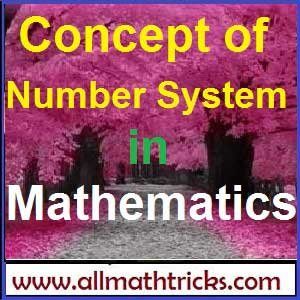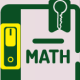# Number System in Mathematics

## The Concepts of number system the mathematics

The number system mainly into classified into 8 types.

1. Complex numbers
2. Imaginary numbers
3. Real numbers
4. Rational numbers
5. Irrational numbers
6. Integers
7. Whole numbers
8. Natural numbers

#### 1. Complex numbers :

Every number in number system taken as a complex number

#### 2. Imaginary Number :

A number does not exist in the number line is called imaginary number. For example square root of negative numbers are imaginary numbers. It is denoted by ” i ” or ” j “

i.e √-1 = i

i2 = – 1

So there is no real number i that satisfies the above equation.  The quantity ” i” is called the unit imaginary number.

#### 3. Real numbers :

All numbers that can be represented on the number line are called real numbers.

The real numbers is the set of numbers containing all of the rational numbers and all of the irrational numbers. The real numbers are “all the numbers” on the number line.

Real Numbers are denoted by “R”.

#### 3. Rational numbers :

A rational number is defined as number of the form x/y where x and y are integers and Y # 0.

i.e Any number which can be expressed as in the form of p/q where “p” and “q” are the integers and q # 0

The set of rational numbers encloses the set of integers and fractions.

The rational numbers that are not integral will have decimal values. These values can be of two types

Terminating decimal fractions (finite decimal factors): For example 1/5 = 0.5 , 13/5 = 2.6.

Non Terminating decimal fractions : The non terminating decimal fractions having two types.

• Non terminating periodic fractions
• Non terminating non periodic fractions

Non terminating periodic fractions : These are non terminating decimal fractions of the type   a. b1b2b3b4b…..bmb1b2b3b4b…..bm.

19/6 = 3.16666666…..

18/7 = 2.57142857142857…….

21/9= 2.3333…….

Non terminating non periodic fractions : These are non terminating and there is no periodic decimal places for that number . i.e a. b1b2b3b4b…..bmc1c2………

for example 6.789542587436512……….

So from above terminating and non terminating periodic fraction numbers belongs to rational numbers.

#### 3. Irrational numbers :

An Irrational numbers are non terminating and non periodic fractions. i.e irrational number is a number that cannot be written as a ratio x/y form (or fraction).  In decimal form, is never ends or repeats.

Examples for irrational numbers are √2 = 1.414213……, π = 3.14159265…….,  √3, √5 etc.

#### 4. Integers ( numbers having no decimals )

All numbers that do not having the decimal places in them are called integers.

All whole numbers including Negative number + Positive number

Z = {∞…..-5,-4,-3,-2,-1,0,1,2,3,4,5….∞}

i.e the integer it may positive or negative or zero.

The set of integers generally written  Z for short.

Any integers are added, subtracted, or multiplied the result is always is an integer.

When any integers multiplied , each of the multiplied integer is called a factor or divisor of the resulting product.

#### 5. Whole numbers :

The set of whole numbers means narrator numbers and “0”

Whole numbers = W = { 0,1,2,3,4,5,6,7,8,…………..∞}

#### 6. Natural Numbers:

The counting numbers start with 1 and their end is not defined. Generally it is  denoted by “N”

i.e N ={1,2,3,4 ………………………….∞}

Another Some important number system :

• Negative integers
• Non Negative integers
• Positive integers
• Non positive integers
• Prime numbers
• Composite numbers
• Co prime numbers
• Even and Odd numbers
• Perfect number.

Negative integers:

The integer is less than zero than it is called negative integer.

i.e the set of integers { -1,-2,-3,-4,-5………….∞} is called negative integers.

The product of any two negative integers is always positive integer

Non Negative integers:

The set of numbers Zero and natural numbers  is called non negative integers.

i.e {01,2,3,4 ………………………….∞}

Positive integers:

The integer is greater than zero than it is called positive integer. The set of natural numbers  is called non negative integers.

The product of any two positive integers is always positive integer.

The product of any two positive and negative integers is always negative integer.

i.e the set of numbers {01,2,3,4 ………………………….∞}

Non positive integers:

The integer is less than zero and include zero than it is called non positive integer.

The set of numbers { 0,-1,-2,-3,-4,-5………….∞} is called non positive integers

Prime numbers:

A natural number larger than unity is a prime number and it does not having any other divisors except for unity and itself is called a prime number

For example : 2,3,5,7,11,13,17,19…………..

• “1 ” is not a prime number.
• “2 ” is the only even and lowest prime number.
• Any prime number more than 6 than, it comes reminder 1 or 5 when that number is division by 6.
• Any prime number more than 5 , that prime number square  division by 24  than reminder comes always 1
• If p and q are any two prime numbers than p2+q2 and p2 -q2are composite numbers.
• Prime number exactly having two different factors.
• No. of prime numbers from 1 to 50 = 15
• No. of prime numbers from 1 to 100 =25.
• No. of prime numbers from 1 to 200 =46
• No. of prime numbers from 1 to 1000 =168

Composite numbers:

A number having one more divisor apart from 1 and itself is called composite number.

i.e The composite number having atleast three divisors.

In the mathematical term any composite number ” n ” can be expressed as

n = m1a x m2b x m3c x m4d

Here m1,  m2, m3 , m4, are the prime numbers and a.b,c,d are the natural numbers.

For example 80 = 51 x 24

Co prime numbers:

Co prime numbers means its having the atleast two numbers and its HCF are  “1 ‘

i.e When two or more numbers having no common prime factors apart from the number ” 1″ , they are called co-prime  or relatively prime to each other.

• Two consecutive odd numbers  are always co-prime numbers  ( Ex : 9 & 11 ,  15&17, 21&23 … etc )
• Two prime numbers are always prime numbers  ( Ex : 3& 7, 11&17, …..etc )
• One prime number and another composite number (Such composite number is not a multiple of the prime number ) are always co-prime numbers. This rule exception only for 17 &51. ( Ex : 3 & 16, 4& 9, ….. etc )
• Three or more numbers being co-prime with each other means that all possible pairs of the numbers would be co- prime with each other.  ( Ex: Thus 51, 53 and 55 are co prime each other than the pairs 51&53, 53&55 ,  51&55 are also co-prime numbers)
• Three consecutive odd numbers are all ways being co-prime numbers. ( Ex : 21,23& 25,  31,33&35 ……… etc.)

Even and Odd numbers:

A number which is a multiple of the number “2” than it is called even number. The even number can be represented by “2n” . here n is natural number.

A number which is not multiple of the number “2” than it is called odd number. The odd number can be represented by “2n+1” . here n is natural number.

Operation of Even and Odd numbers:

Odd x Odd = Odd

Odd + Odd = Even

Odd – Odd = Even

Odd /  Odd = Odd

Even + Even = Even

Even x Even = Even

Even – even = Even

Even / Even = Even or Odd

Odd x Even = Even

Odd + Even = Odd

Even/ Odd = Even

Odd / Even = Not divisible.

Perfect number:

Any number  is called perfect number, if the sum of all its factors excepting itself is equal to the number itself .

For example take a numbers 6. Factors of 6 are 1,2,3,6

Sum of the factors except itself = 1+2+3 = 6

So the number ” 6 ” is called perfect number.Rules for Divisibility of numbers

Formulas for Sum of n Consecutive numbers

Methods to find HCF & LCM

GCD and LCM Problems & Solutions

Simple interest and Compound interest formulas

## 6 thoughts on “Number System in Mathematics”

#### Sum of n Consecutive numbers Like Natural, Even, Odd, Squares, Cubes

(November 7, 2017 - 5:53 pm)

[…] this page provide formulas with examples for sum of n consecutive natural numbers, sum of positive integers,  sum of n odd and even numbers, sum of consecutive squares of natural, […]#### Sumit

(October 21, 2018 - 10:28 pm)

I humbly request to you, Provide me concept of all topics of mathematics .#### sivaalluri

(October 25, 2018 - 5:16 pm)

Ok we will provide all topic one by one#### johnson

(September 12, 2019 - 9:11 am)

kindly how can one prove a irrational number is no a rational number#### ABC

(April 13, 2020 - 4:34 pm)

If these are types of Number System then….
What are these…Octal, Decimal number system etc..?
I mean,These are also type of Number system.?#### ABC

(April 13, 2020 - 4:36 pm)